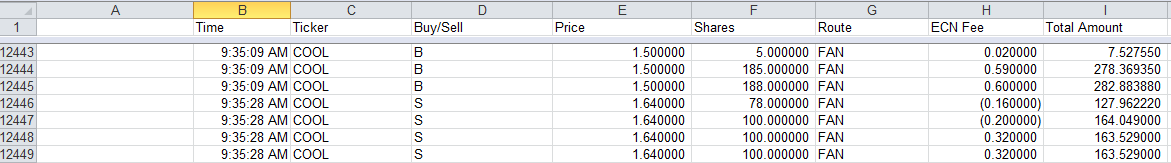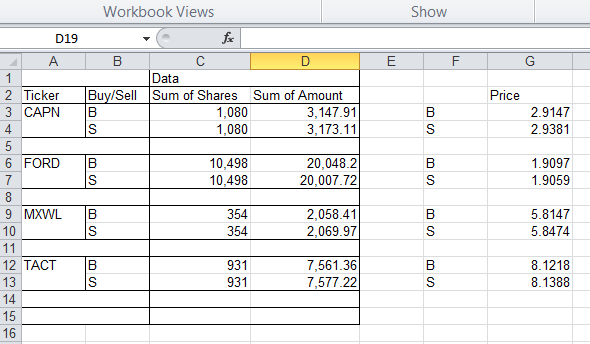First, start with how the data should be formatted prior to the macro working. Go to “Trade” in DAS Trader Pro and then click “Trades”. Select the columns and copy and paste into an Excel worksheet named “scratchpad” (and make sure to paste into column B). Then hit Control + A to select the entire range of data and run the macro (I have it hotkeyed on my computer to Control+P). This will create a new worksheet, run a pivot table, copy the pivot table data, delete the pivot table worksheet, and paste the pivot table data and price per share in an easy to read format in another new worksheet.

Here is how the data should be formatted in your trade report that you paste into Excel (you do not need a header row):And below is how the data will be output:Obviously, you can use this same basic code framework to get lots of other data easily (such as ECN fees, etc). The one thing this code will not do is account for per-trade fees. I don’t have them at Centerpoint (clearing through ETC) so I didn’t program them. Also, make sure you enter your per-share commission into the code. Use this code at your own risk — I provide it without warranty or support.

Warning: if you are a programmer you will find my code ugly. You have been warned.

Below is the code:

‘ For this to work you need to copy pasta the following columns from DAS into the ‘sheet ‘scratchpad’
‘Time | Ticker | Buy/Sell | Price | Shares | Route | ECN Fees | Amount of trade
‘Those columns all must be there in that order or it will mess lots of things up.
‘The trades must be pasted into column B and then select all the trade info ‘(control+A)

Dim myRange As Range
Dim i As Long
Dim TopLeft As Range
Dim objTable As PivotTable
Dim objField As PivotField
Dim rng As Range
Dim ws1 As Worksheet
Dim pt As PivotTable
Dim rngPT As Range
Dim rngPTa As Range
Dim rngCopy As Range
Dim rngCopy2 As Range
Dim lRowTop As Long
Dim lRowsPT As Long
Dim lRowPage As Long
Dim PivotTableSheet As String
Dim celltxt As String

‘this is my commission rate
commission = 0.0035

Set myRange = Selection

‘sort by column C then D (ticker then buy/sell)
myRange.Sort Key1:=Columns(3), Order1:=xlAscending, Key2:=Columns(4) _
, Order2:=xlAscending, Header:=xlGuess, OrderCustom:=1, MatchCase:= _
False, Orientation:=xlTopToBottom
‘the above works! Yay!

‘this section calculates the cost basis (including ECN fees and commissions) of each fill
For i = myRange.Rows.Count To 1 Step -1
myRange.Cells(i, 8) = (myRange.Cells(i, 4) + commission) * myRange.Cells(i, 5) + myRange.Cells(i, 7)
myRange.Cells(i, 8) = (myRange.Cells(i, 4) – commission) * myRange.Cells(i, 5) – myRange.Cells(i, 7)
myRange.Cells(i, 8) = (myRange.Cells(i, 4) – commission) * myRange.Cells(i, 5) – myRange.Cells(i, 7)
End If
Next i

‘Here we append the header row to the data
myRange.Cells(0, 1) = “Time”
myRange.Cells(0, 2) = “Ticker”
myRange.Cells(0, 4) = “Price”
myRange.Cells(0, 5) = “Shares”
myRange.Cells(0, 6) = “Route”
myRange.Cells(0, 7) = “ECN Fee”
myRange.Cells(0, 8) = “Amount”

‘Pivot table time!

‘only uncomment the following two lines when testing on specific region
‘Range(“B4”).Select

myRange.Cells(0, 1).Select

Set objTable = ActiveSheet.PivotTableWizard

Set objField = objTable.PivotFields(“Ticker”)
objField.Orientation = xlRowField
objField.Position = 1

objField.Orientation = xlRowField
objField.Position = 2

Set objField = objTable.PivotFields(“Shares”)
objField.Orientation = xlDataField
objField.Position = 1
objField.Function = xlSum
objField.NumberFormat = “##,###”

Set objField = objTable.PivotFields(“Amount”)
objField.Orientation = xlDataField
objField.Position = 2
objField.Function = xlSum
objField.NumberFormat = “##,###.##”

‘Pivot tables with multiple data fields have hidden field “data” —
‘adding the below line makes it display correctly

‘Copy the Pivot table and paste it into a new worksheet as values
On Error Resume Next
Set pt = ActiveCell.PivotTable
Set rngPTa = pt.PageRange
‘On Error GoTo errHandler

PivotTableSheet = ActiveSheet.Name

‘If pt Is Nothing Then
‘ MsgBox “Could not copy pivot table for active cell”
‘ GoTo exitHandler
‘Else
Set rngPT = pt.TableRange1
lRowTop = rngPT.Rows(1).row
lRowsPT = rngPT.Rows.Count
Set rngCopy = rngPT.Resize(lRowsPT – 1)
Set rngCopy2 = rngPT.Rows(lRowsPT)

rngCopy.Copy Destination:=ws1.Cells(lRowTop, 1)
rngCopy2.Copy Destination:=ws1.Cells(lRowTop + lRowsPT – 1, 1)
‘End If

If Not rngPTa Is Nothing Then
lRowPage = rngPTa.Rows(1).row
rngPTa.Copy Destination:=ws1.Cells(lRowPage, 1)
End If

ws.Columns.AutoFit

‘delete pivot table sheet
Sheets(PivotTableSheet).Delete

Columns(“C:C”).ColumnWidth = 13.71
Columns(“D:D”).ColumnWidth = 14.86
Range(“G2”).Select
ActiveCell.FormulaR1C1 = “Price”
Columns(“G:G”).Select
Selection.NumberFormat = “0.0000”
Selection.ColumnWidth = 11.43
Range(“F3”).Select
ActiveCell.FormulaR1C1 = “=IF(ISBLANK(RC[-4]),””””,RC[-4])”
Range(“F3”).Select
Selection.AutoFill Destination:=Range(“F3:F197”), Type:=xlFillDefault
Range(“F3:F197”).Select
Range(“G3”).Select
ActiveCell.FormulaR1C1 = _
“=IF(ISBLANK(RC[-3]),””””,IF(ISBLANK(RC[-5]),””””,RC[-3]/RC[-4]))”
Range(“G3”).Select
Selection.AutoFill Destination:=Range(“G3:G202”), Type:=xlFillDefault

‘Loop through table to clear all rows that contain “Total”
Last = Cells(Rows.Count, “D”).End(xlUp).row
For i = Last To 1 Step -1
celltxt = Cells(i, “A”).Text
If InStr(1, celltxt, “Total”) Then
Cells(i, “A”).EntireRow.ClearContents ‘ USE THIS TO CLEAR CONTENTS BUT NOT DELETE ROW
End If
Next i

ActiveSheet.UsedRange.Select

exitHandler:
Exit Sub
errHandler:
MsgBox “Could not copy pivot table for active cell”
Resume exitHandler
End Sub

Disclaimer: I have no position in any stocks mentioned as of this post being published but I may trade them in the future. I am a client of Centerpoint Securities (clearing through ETC). I have no relationship with any other parties mentioned above. This blog has a terms of use that is incorporated by reference into this post; you can find all my disclaimers and disclosures there as well.

1.Dario says: